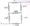# Basic Electrical Engineering May 2009

UNIT-I

Q.1 (a) Why are electrical machine made of magnetic materials?

(b) State and explain super position theorem.

(c) Determine the equivalent resistance between the terminals X and Y of the circuit shown.

(d) A steel ring of 25 cm in diameter and of circular section 3 cm in diameter has an air gap of 1.5 mm length. If is would uniformly with 750 turns of wire carrying a current of 2.1 A.

Calculate:

(i) Magneto motive force.

(ii) Flux density in air gap.

(iii) Magnetic flux.

(iv) Relative permeability of steel ring.

Assume that iron path takes about 35% of the total miff.UNIT-II

Q.2 (a) Show that power consumed in a 1 – ?.

Ac circuit is-given by VI coos ?.

(b) Derive the expression for the band width in series RLC circuit.

(c) A circuit consist of R = 35 ohms in series with an unknown impedance Z. For a sinusoidal current of 2 A, the observed voltages are 200 volte across R and z together , 150 volts across impendence Z. Find the impedance Z.

(d) Show that: tan ?(W2 – W1)

W1 + W2

Unit – III

Q.3 (a) The efficiency of a transformer is high compared to other electrical machines. Give reasons .

(b) Write short note on no load and short circuit tests.

(c) What do you mean by efficiency of a transformer ? Derive the condition for maximum efficiency.

(d) A 200 kava transformer has an efficiency of 98% at full load. If the maximum efficiency occurs at 75% of lull load, calculate the efficiency at half load. Assume negligible magnetizing current and pf at 0.8 atall load.

UNIT-IV

Q.4 (a) A series motor should never be started with our load on if Give reasons .

(b) A 120 V decent motor has an armature resistance of 02 ohrr and a field resistance of 60 ohms. It runs at 1800 rpm it is taking a full load current of 40A. Find the speed of the moto when it is operating at half full load.

(c) With usual notations, derive the torque equation of a 0.1 motor.

(d) Derive the expression for developed torque in a 3 phase in diction motor.

UNIT-V

Q.5 (a) How do you extend the rate of an ammeter?

(b) What is the principle of a moving iron voltmeter?

(c) Explain the operation of a single phase energy meter.

(d) A single phase K who meter makes 500 revolutions per K Who. It is found in testing as making 40 revolutions in 58.1 seconds at 5 kW full load find out the percentage error.